Q-Chem 5.0 User’s Manual

# 12.8 The Adiabatic ALMO-EDA Method

Despite the huge success and usefulness of today’s most popular EDA methods, they still face some limitations in their capabilities. For instance, EDAs are usually performed at complex geometries that are obtained from unconstrained electronic structure calculations (e.g. optimized equilibrium geometries). For strongly interacting systems, close intermolecular contacts driven by POL and particularly CT often result in largely unfavorable FRZ interaction, which offers little physical insights besides indicating obviously substantial intermolecular overlap. Another limitation is that the conventional EDA methods often partitions a “single-point" interaction energy evaluated at a given geometry. Therefore, the influence of FRZ, POL and CT on the structural and vibrational properties of an intermolecular complex cannot be directly characterized.

Recently Mao et al. reformulated the original ALMO-EDA method in an “adiabatic" picture . The term “adiabatic" is borrowed from spectroscopy, which indicates that the energy differences are evaluated at relaxed geometry on each potential energy surface (PES). In this scheme, the total binding energy (including monomer geometry distortions) is repartitioned into adiabatic FRZ, POL and CT terms: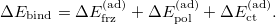(12.13)

The adiabatic frozen interaction energy is given by the difference between the energy minimum of the frozen PES (on which the energy of each point is computed using the corresponding frozen wave function) and the sum of fully relaxed, non-interacting fragment energies: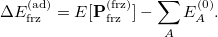(12.14)

Similarly, the adiabatic POL and CT terms can be obtained by performing geometry optimizations on the polarized (SCFMI) and fully relaxed (unconstrained SCF) PESs: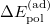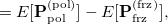(12.15)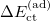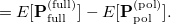(12.16)

With this method, the changes in monomer structures and intermolecular coordinates due to FRZ, POL and CT and the accompanied energetics are provided. Moreover, at the energy minimum (or other stationary points) on each PES, the other properties such as multipole points, vibrational frequencies and intensities can also be computed, therefore the effect of different intermolecular interaction components on them can also be characterized.

The geometry optimization on the frozen PES is facilitated by the analytical gradient of the frozen wave function energy implemented in Q-Chem. As for the geometry optimization on the polarized PES, the nuclear gradient of the SCFMI energy has the same form as that of the full SCF energy if the original ALMO model is utilized. These analytical gradients can also be used for finite difference calculations of harmonic frequencies by setting IDERIV = 1. We note that the analytical gradients of SCFMI calculations that use FERFs are not available yet, and SCFMI_MODE = 0 is required for computing the forces on the frozen and polarized PESs. Also, the current implementation of this method requires users to perform geometry optimization on the three PESs separately (see the example below) and evaluate the energy components by taking several Q-Chem outputs (including geometry optimizations for the monomers) together, which is probably not so convenient. We look forward to extending the functionality of this method and improving its implementation in the near future.

FRZ_GEOM
 Compute forces on the frozen PES.

TYPE:
 BOOLEAN

DEFAULT:
 FALSE

OPTIONS:
 FALSE Do not compute forces on the frozen PES. TRUE Compute forces on the frozen PES.

RECOMMENDATION:
 Set it to TRUE when optimized geometry or vibrational frequencies on the frozen PES are desired.

POL_GEOM
 Compute forces on the polarized (converged SCFMI) PES.

TYPE:
 BOOLEAN

DEFAULT:
 FALSE

OPTIONS:
 FALSE Do not compute forces on the polarized PES. TRUE Compute forces on the polarized PES.

RECOMMENDATION:
 Set it to TRUE when optimized geometry or vibrational frequencies on the polarized PES are desired.

Example 12.313  Geometry optimization of the ammonia-borane complex on the fully relaxed, polarized, and frozen potential energy surfaces successively.

$molecule 0 1 -- 0 1 H 0.000000 0.000000 0.000000 H 0.000000 0.000000 1.629090 H 1.417687 0.000000 0.814543 N 0.473683 -0.370067 0.814542 -- 0 1 N 3.494032 -1.531250 0.814538 H 3.967715 -1.901317 -0.000008 H 2.550028 -1.901319 0.814537 H 3.967715 -1.901317 1.629083$end

$rem JOBTYPE opt !optimization on the fully relaxed PES GEN_SCFMAN true METHOD wb97x-d BASIS 6-31+g* XC_GRID 1 THRESH 14 SCF_CONVERGENCE 9 SCF_GUESS fragmo SYMMETRY false SYM_IGNORE true$end

@@@

$molecule read$end

$rem JOBTYPE opt POL_GEOM true !optimization on the polarized PES GEN_SCFMAN true METHOD wb97x-d BASIS 6-31+g* XC_GRID 1 THRESH 14 SCF_CONVERGENCE 9 SYMMETRY false SYM_IGNORE true SCFMI_MODE 0$end

@@@

$molecule read$end

$rem JOBTYPE opt FRZ_GEOM true !optimization on the frozen PES GEN_SCFMAN true METHOD wb97x-d BASIS 6-31+g* XC_GRID 1 THRESH 14 SCF_CONVERGENCE 9 SYMMETRY false SYM_IGNORE true SCFMI_MODE 0$end


Example 12.314  Geometry optimization of the CFHClcomplex on the frozen PES followed by a calculation for harmonic frequencies at the equilibrium geometry.

$molecule -1 1 -- 0 1 C 0.000000 0.000000 0.905509 H 0.000000 0.000000 -0.179815 F 1.250779 0.000000 1.406437 F -0.625389 -1.083207 1.406437 F -0.625389 1.083207 1.406437 -- -1 1 Cl 0.000000 0.000000 -2.664391$end

$rem JOBTYPE opt FRZ_GEOM true GEN_SCFMAN true METHOD wb97X-V BASIS def2-svpd XC_GRID 000075000302 THRESH 14 SCF_CONVERGENCE 9 SYMMETRY false SYM_IGNORE false SCFMI_MODE 0 GEOM_OPT_TOL_GRADIENT 100 GEOM_OPT_TOL_DISPLACEMENT 100 GEOM_OPT_TOL_ENERGY 10 FD_MAT_VEC_PROD false$end

@@@

$molecule read$end

$rem JOBTYPE freq FRZ_GEOM true GEN_SCFMAN true METHOD wb97X-V BASIS def2-svpd XC_GRID 000075000302 THRESH 14 SCF_CONVERGENCE 9 SYMMETRY false SYM_IGNORE false IDERIV 1 SCFMI_MODE 0 FD_MAT_VEC_PROD false$end